• +91 9971497814
• info@interviewmaterial.com

# RD Chapter 23- The Straight Lines Ex-23.11 Interview Questions Answers

### Related Subjects

Question 1 :
Prove that the following sets of three lines are concurrent:
(i) 15x – 18y + 1 = 0, 12x + 10y – 3 = 0 and 6x + 66y – 11 = 0
(ii) 3x – 5y – 11 = 0, 5x + 3y – 7 = 0 and x + 2y = 0

(i) 15x – 18y + 1 = 0, 12x+ 10y – 3 = 0 and 6x + 66y – 11 = 0

Given:

15x – 18y + 1 = 0 ……(i)

12x + 10y – 3 = 0 ……(ii)

6x + 66y – 11 = 0 ……(iii)

Now, consider thefollowing determinant: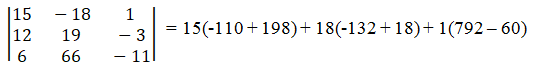=> 1320 – 2052 +732 = 0

Hence proved, thegiven lines are concurrent.

(ii) 3x – 5y – 11 = 0, 5x +3y – 7 = 0 and x + 2y = 0

Given:

3x − 5y − 11= 0 …… (i)

5x + 3y − 7= 0 …… (ii)

x + 2y =0 …… (iii)

Now, consider thefollowing determinant: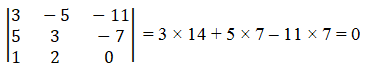Hence, the given linesare concurrent.

Question 2 : For what value of λ are the three lines 2x – 5y + 3 = 0, 5x – 9y + λ = 0 and x – 2y + 1 = 0 concurrent?

Given:

2x − 5y + 3= 0 … (1)

5x − 9y+ λ = 0 … (2)

x − 2y + 1 =0 … (3)

It is given that thethree lines are concurrent.

Now, consider thefollowing determinant: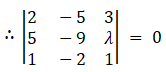2(-9 + 2λ) + 5(5– λ) + 3(-10 + 9) = 0

-18 + 4λ + 25 –5λ – 3 = 0

λ = 4

The value ofλ is 4.

Question 3 :

Find the conditions that the straight lines y = m1x + c1,y = m2x + c2 and y = m3x + c3 maymeet in a point.

Given:

m1x – y + c1 =0 … (1)

m2x – y + c2 =0 … (2)

m3x – y + c3 =0 … (3)

It is given that thethree lines are concurrent.

Now, consider thefollowing determinant: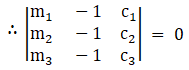m1(-c3 +c2) + 1(m2c3-m3c2) + c1(-m2 +m3) = 0

m1(c2-c3)+ m2(c3-c1) + m3(c1-c2)= 0

The requiredcondition is m1(c2-c3) + m2(c3-c1)+ m3(c1-c2) = 0

Question 4 :

If the lines p1x + q1y = 1, p2x + q2y= 1 and p3x + q3y = 1 be concurrent, show that the points(p1, q1), (p2, q2) and (p3,q3) are collinear.

Given:

p1x + q1y= 1

p2x + q2y= 1

p3x + q3y= 1

The given lines can bewritten as follows:

p1 x +q1 y – 1 = 0 … (1)

p2 x +q2 y – 1 = 0 … (2)

p3 x +q3 y – 1 = 0 … (3)

It is given that thethree lines are concurrent.

Now, consider thefollowing determinant: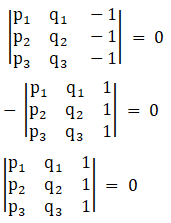Hence proved, thegiven three points, (p1, q1), (p2, q2)and (p3, q3) are collinear.

Question 5 :

Show that the straight lines L1 = (b + c)x + ay + 1 = 0,L2 = (c + a)x + by + 1 = 0 and L3 = (a + b)x +cy + 1 = 0 are concurrent.

Given:

L1 =(b + c)x + ay + 1 = 0

L2 =(c + a)x + by + 1 = 0

L3 =(a + b)x + cy + 1 = 0

The given lines can bewritten as follows:

(b + c) x + ay + 1 = 0… (1)

(c + a) x + by + 1 = 0… (2)

(a + b) x + cy + 1 = 0… (3)

Consider the followingdeterminant.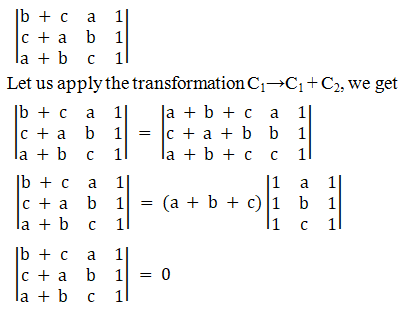Hence proved, thegiven lines are concurrent.

Todays Deals### RD Chapter 23- The Straight Lines Ex-23.11 Contributorskrishan

Name:
Email:

# Latest News# 9000 interview questions in different categories